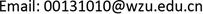1. 引言

2. 鞋底发电装置整体设计2.1. 整体设计方案

2.2. 运行原理

3. 鞋底发电装置关键零部件设计3.1. 齿轮齿条设计

d = m z = 0.8 × 10 = 8   mm (1)

D a = m ( z + 2 ) = 0.8 × 12 = 9.6   mm (2)

p = π m = 3.14 × 0.8 = 2.512   mm (3)

H = d 2 + x m = 4.56   mm (4)

m t = m cos β = 0.8 cos 8 ∘ ≈ 0.81   mm (5)

P n = π m n = 3.14 × 0.81 = 2.54   mm (6)

3.2. 增速齿轮组设计

d = m z (8)

3.3. 棘轮棘爪设计

3.3.1. 棘轮轮齿模数齿数的确定

m = d a z (9)

h = 0.75 m (10)

d f = d a − 2 h = d a − 1.5 m (11)

3.3.2. 棘爪设计

l = π m (12)

h 1 = h + ( 2 − 3 ) m m (13)

h1 = 3.875 mm。

a1 = 2.5 mm。

4. 测试

5. 总结